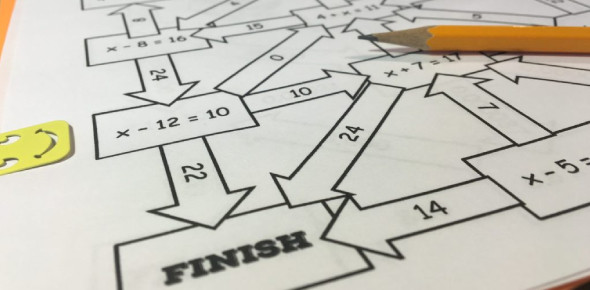# IQ Test For 6th Graders

12 Questions | Total Attempts: 22693SettingsAre you ready for this IQ test for 6th graders to see how much do you know? In this quiz, you will come across some questions that will test your math knowledge of 6th grade. If you manage to get a score equal to or above 80, then your 6th-grade math IQ is high. Below 80 and above 50 means average, and below 50 means low. Let's go for it!

• 1.
If a = 1.9 and b = 0.6, what is a/b (round to 2 decimal places)?
• A.

0.32

• B.

2.5

• C.

3.17

• D.

1.3

• 2.
Which step is 2nd when you use the Order of Operations Agreement?
• A.

Multiplication and Division

• B.

Grouping

• C.

• D.

Exponents

• 3.
How much is 1/4 of the sum of 260 and 64?
• A.

324

• B.

16

• C.

65

• D.

81

• 4.
Which number is evenly divisible by 7?
• A.

674

• B.

672

• C.

673

• D.

671

• 5.
What is 2/3 of 192?
• A.

108

• B.

118

• C.

138

• D.

128

• 6.
How many feet are in 2/3 of a mile?
• A.

1,760 Feet

• B.

8,520 Feet

• C.

5,280 Feet

• D.

3,520 Feet

• 7.
In how many different ways can the cars of a three-car train be arranged?
• A.

Six

• B.

10

• C.

30

• D.

20

• 8.
What's the width of a cube whose height is 10 inches?
• A.

20 Inches

• B.

10 Inches

• C.

100 Inches

• D.

5 Inches

• 9.
How would you write 4^3 (4 to the 3rd power) without an exponent?
• A.

16

• B.

64

• C.

12

• D.

43

• 10.
Which of the examples below shows the "Multiplicative Inverse"?
• A.

2 + (-2) = 0

• B.

2 x (1/2) = 1

• C.

2 + 0 = 2

• D.

2 x 0 = 0

• 11.
What is the prime factorization of 100?
• A.

10 x 10

• B.

2 x 2 x 5 x 5

• C.

2 x 2 x 25

• D.

2 x 5

• 12.
Which step is first when using the order of operations?
• A.

• B.

Grouping

• C.

Exponents

• D.

Multiplication and Division

Related TopicsBack to top